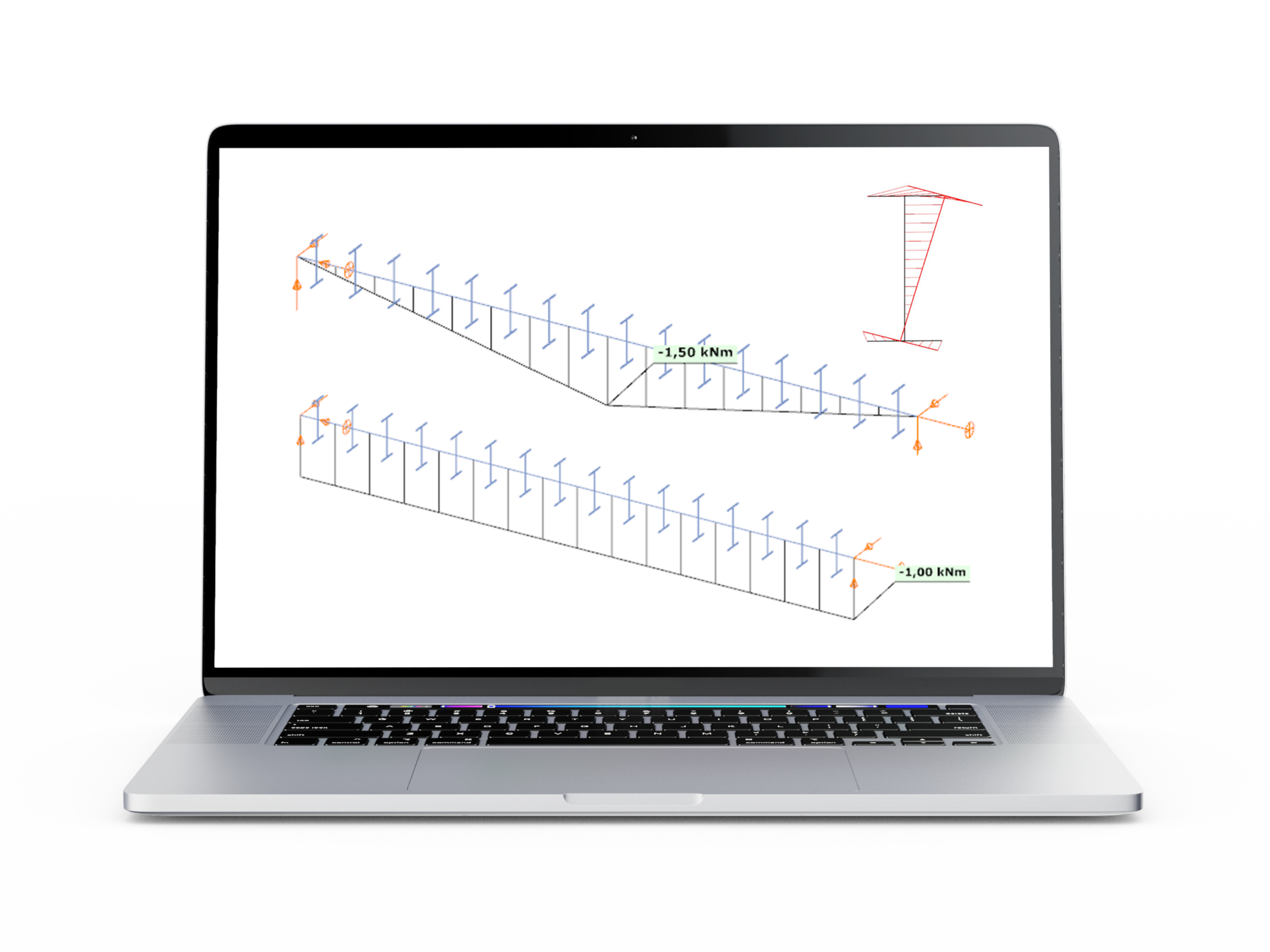EN# Lateral torsional buckling of a member with doubly symmetric section - Verification example

## Introduction

This verification example studies a simple fork supported beam member with welded section (flanges: 200-12; web: 400-8) subjected to bending about major axis. Constant bending moment due to concentrated end moments and triangular moment dsitribution from concentrated transverse force is examined. Critical moment and force of the member is calculated by hand and by the Consteel software using both 7 DOF beam finite element model and Superbeam function.

## Geometry

Constant bending moment distribution

Triangular bending moment distribution – load on upper flange

Triangular bending moment distribution – load on bottom flange

## Calculation by hand

Constant bending moment distribution

Triangular bending moment distribution

## Computation by Consteel

Version nr: Consteel 15 build 1722

Constant bending moment distribution

7 DOF beam element

First buckling eigenvalue of the member which was computed by the Consteel software using the 7 DOF beam finite element model (n=16). The eigenshape shows lateral torsional buckling.

Superbeam

First buckling eigenvalue of the member which was computed by the Consteel software using the Superbeam function (δ=25).

Triangular bending moment distribution – load on upper flange

7 DOF beam element

First buckling eigenvalue of the member which was computed by the Consteel software using the 7 DOF beam finite element model (n=16).

Superbeam

First buckling eigenvalue of the member which was computed by the Consteel software using the Superbeam function (δ=25).

Triangular bending moment distribution – load on bottom flange